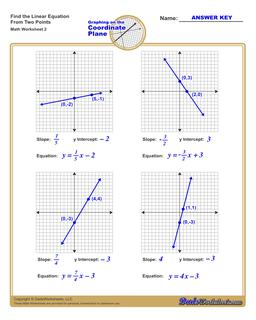One Dad. Four daughters. 9,341 worksheets... and counting!# Find the Equation of a Line (Second Worksheet)

PropertyValue
DescriptionFind the Equation of a Line: Each linear equations worksheet on this page shows four graphs on a coordinate plane, each with two points labeled, and students find the equation in slope-intercept form by calculating both the slope and y-intercept. (Second Worksheet)
Resource TypeWorksheet